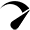# VEDIC MATH ONLINE CLASSES

An ancient system of mathematics, Vedic Math is based on simple rules and principles through which mathematical problem can be solved mentally. Vedic math is a set of sixteen Sanskrit mathematical formulae or sutras and their corollaries derived from the Vedas, which were formulated over centuries. The Sutras were lost over time but thankfully, re- discovered by Jagadguru Swami Sri Bharati Krisna Tirthaji Maharaja between 1911 and 1918.

Vedic Mathematics is speed friendly and it is the simplest, the fastest and the purest form of Mathematics. Slowly and slowly, the world is realizing this fact. The Sutras of Vedic Math are very helpful in understanding many facts of the number theory. The fast calculation techniques are one of the greatest tools to enhance the ability to do the question at the required speed and also to avoid big negative marking punishment in the math score.

Vedic Mathematics was not known to the world but with an increase of interest in ancient Sanskrit text, the ancient Vedic Mathematics was rediscovered by Swami Bharati Krisna Tirthaji (the former Shankaracharya of Puri, India) in 1911. Swami Bharati Krisna Tirthaji was a great scholar of Sanskrit, Mathematics, History and Philosophy. His deep study and careful research had deciphered the great mathematical formulas known as Sutras that were completely ignored as no one could relate these to mathematics.

Vedic Mathematics written in 1965, that is a pioneer work of Bharati Krishna Tirthaji, has techniques of Vedic mathematics. It is considered as a first work towards Vedic Mathematics.

In late 1960s a copy of his book reached London and from there Vedic Mathematics was reborn. It was taken up as a new alternative system of mathematics. British mathematicians got interested in Vedic mathematics. Many lectures on this were delivered which later on were collected in the book – Introductory Lectures on Vedic Mathematics. After the visit of Andrew Nicholas to India between 1981 and 1987, interest in Vedic mathematics started to develop in India.

Vedic mathematics has given a new approach to mathematics. To do calculations quickly, the Indian Institute of Technology IIT students are said to use Vedic Mathematics. Without a doubt there are many advantages of learning Vedic Mathematics. Students develop problem solving ability and it also leads to the development of creative intelligence. Students of Vedic Mathematics can not only do simple calculations of subtraction, addition, multiplication but also very complex calculations such as algebra, geometry, calculus and trigonometry. Their mind is at work with this mathematics so mental sharpness is associated with it.

Vedic mathematics is very effective and at the same time it is easy to learn. Only 16 Vedic sutras are used to solve the mathematical problem. Sutras are basically word formulae that describe normal way of solving mathematical problems.

Instead of learning by repetition, Vedic Mathematics involves logic and understanding the fundamental concepts. One can do calculations much faster than done by using the conventional method that is taught in schools. It teaches the students to solve same problem in different ways.

Now, the question is if India has such a wonderful and useful way of calculating mathematical problems then why it is not taught in all the school, right from the beginning. Why we rely just on Western education pattern and learning through logical reasoning instead of mentally solving them. People must be encouraged to learn Vedic mathematics and it must be part of our curricula.

Vedic Mathematics is speed friendly and it is the simplest, the fastest and the purest form of Mathematics. Slowly and slowly, the world is realizing this fact. The Sutras of Vedic Math are very helpful in understanding many facts of the number theory. The fast calculation techniques are one of the greatest tools to enhance the ability to do the question at the required speed and also to avoid big negative marking punishment in the math score.Optimized by Seraphinite Accelerator
Turns on site high speed to be attractive for people and search engines.